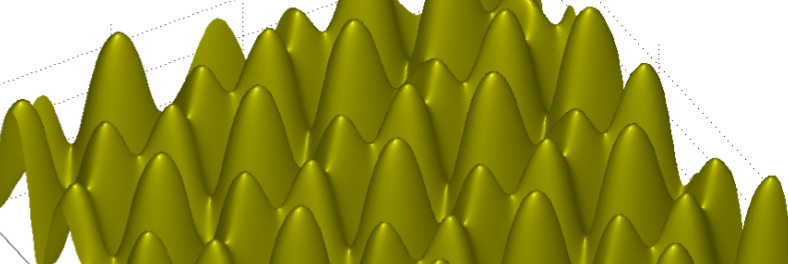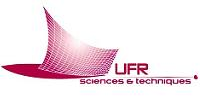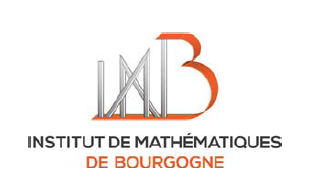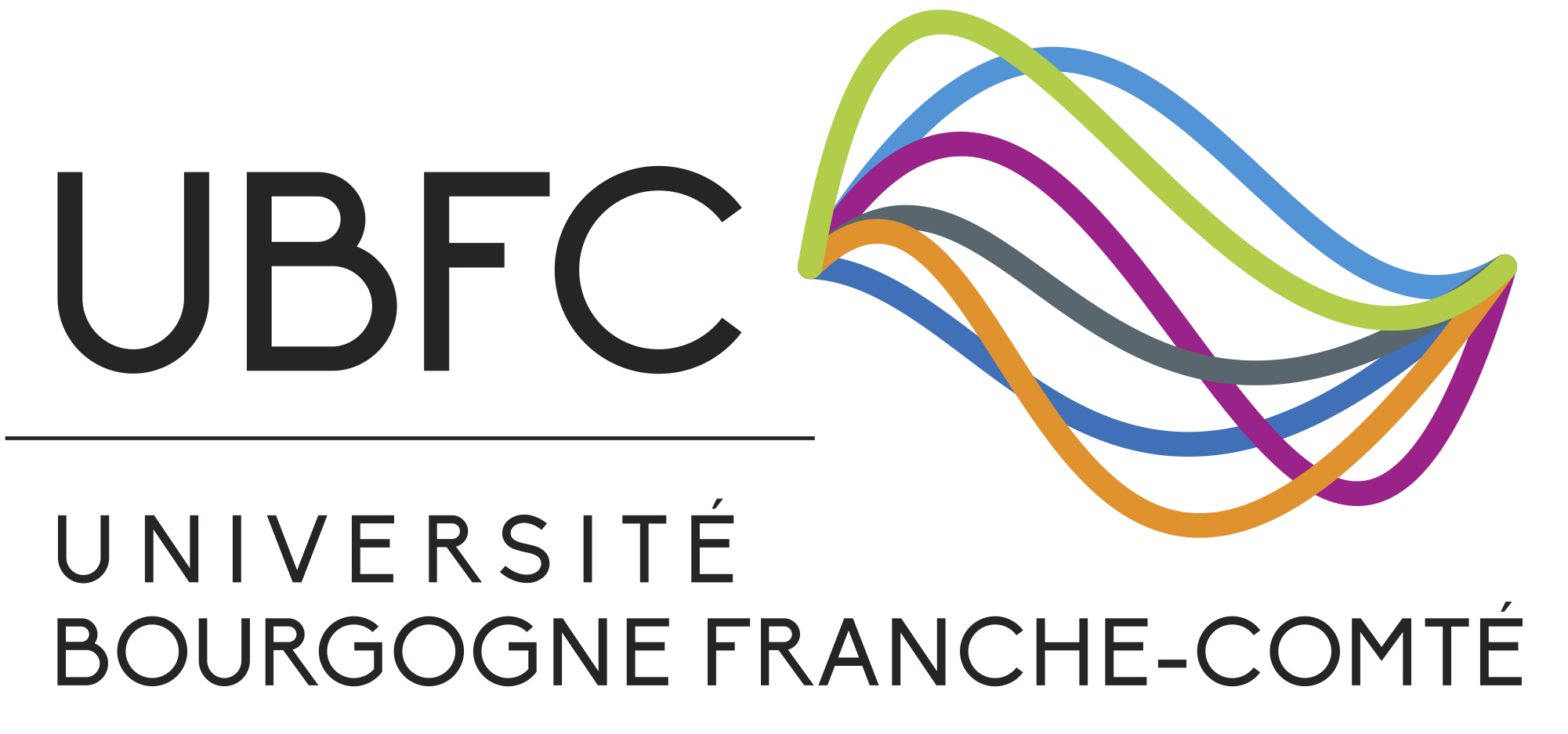# Master program in Mathematical Physics

### Mathematical Methods of modern Theoretical Physics

Consult the new website of the master program.

The Department of Mathematics of the Université de Bourgogne (Dijon, France) opens a two-years master program in Mathematical Physics. The main aim of this program is to provide advanced lectures on the mathematical methods of modern theoretical physics in the framework of mathematics cursus. We offer lecture courses for the students with background in mathematics or mathematical physics giving an introduction to the mathematical methods used for such branches of theoretical physics as quantum field theory, statistical mechanics, general relativity, gauge theories, string theory etc. The coursework will cover different fields of mathematics (algebra, geometry, analysis) and will highlight their applications to the problems of theoretical physics.  The student will be integrated from the very beginning into the mathematical physics group of the IMB (Burgundy Mathematical Institute) and will have to prepare by the end of the year a  masters dissertation. All the lectures will be given in English

 The lecture coursework of the second year include: Mathematical methods of quantum physics (N. Kitanine) Riemann geometry and integrable systems  (G. Carlet) Lie groups and Lie algebras (G. Dito) Quantum groups (P. Schauenburg) Cohomological field theories* (Does not open 2018/2019) Geometry and physics of black hole spacetimes (J.L. Jaramillo)

We will also provide several mini courses by the research visitors of IMB as well as by  invited professors in the framework of the Erasmus program.

Information for the 2018/2019 students:

Information for prospective 2019/2020 students:

Contact: Nikolai Kitanine (Nikolai.Kitanine at u-bourgogne.fr)